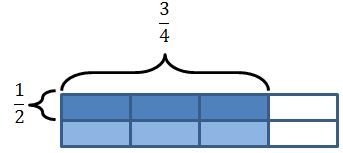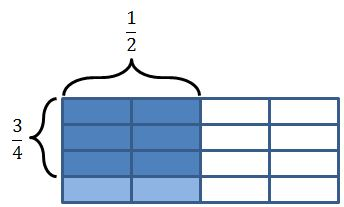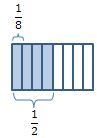• If you are citizen of an European Union member nation, you may not use this service unless you are at least 16 years old.

View

# 6-N-4-2

last edited by 2 years, 7 months ago

6.N.4.2 Illustrate multiplication and division of fractions and decimals to show connections to fractions, whole number multiplication, and inverse relationships.

In a Nutshell

Visual models such as number lines and fraction strips can be used to model any problem involving multiplication and division of fractions. The rectangular area model used to model whole number multiplication in earlier grades is an additional model that can be used to model fraction multiplication. The inverse relationship between multiplication and division is best illustrated using the number line or fraction strip model because the model used for a given multiplication problem will be the same as the model used for the related division problem. Decimal multiplication and division can also be illustrated visually using models such as number lines, base 10 blocks, and hundredths grids. When illustrating decimal multiplication using base 10 blocks or hundredths grids, the products that can be shown are limited to products that have numbers in place-values up to the hundredths place.  The purpose of illustrating multiplication and division of fractions and decimals is to provide the opportunity to gain a deeper understanding of the underlying algorithms for these operations.

## Teacher Actions

• Develop the ability to model and draw conclusions about multiplying and dividing fractions using a variety of models, such as number lines and fraction strips. Rectangular area models can also be used to show the connection between multiplication of whole numbers and multiplication of fractions.

• Develop the ability to model and draw conclusions about multiplying and dividing decimals using a variety of models, such as number lines, base 10 blocks or hundredths grids.

• Develop a deep and flexible understanding of multiplication and division by using models to show that multiplication and division are inverse operations.

• Use and connect mathematical representations between the students' previous experiences with modeling multiplication and division of whole numbers to modeling multiplication and division of fractions and decimals.

• Use and connect mathematical representations between the models of multiplication and division of fractions and decimals.

• Pose purposeful questions to assess a student’s progress towards understanding that multiplication and division are inverse operations and adjust instruction to support their understanding. (Ex. Using the fraction strip model to support your answer,  how does fraction multiplication compare to fraction division?)

## Misconceptions

• Understand fraction multiplication and division can be illustrated visually with models such as number lines and fraction strips.

• Understand decimal multiplication and division can be illustrated visually with models such as number lines.

• Understand multiplication and division are inverse operations.

• Struggle to make meaning of problems involving fractions, such as 1/21 3/4 and 51/4, making it difficult to assess reasonableness of results.

• Not recognize equivalencies when comparing two rectangular area models.  For example, students may think the following area models for 1/23/4 which result in a product of 3/8 do not represent the same multiplication problem.• Think the quotient of 1/21/8 is 4/8 instead of the correct answer which is 4 when modeling the division problem using the fraction strip model.OKMath Framework Introduction